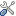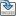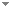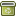# The length of some diagonalization games

Archive for Mathematical Logic 38 (2):103-122 (1999)

 Abstract For X a separable metric space and $\alpha$ an infinite ordinal, consider the following three games of length $\alpha$ : In $G^{\alpha}_1$ ONE chooses in inning $\gamma$ an $\omega$ –cover $O_{\gamma}$ of X; TWO responds with a $T_{\gamma}\in O_{\gamma}$ . TWO wins if $\{T_{\gamma}:\gamma<\alpha\}$ is an $\omega$ –cover of X; ONE wins otherwise. In $G^{\alpha}_2$ ONE chooses in inning $\gamma$ a subset $O_{\gamma}$ of ${\sf C}_p(X)$ which has the zero function $\underline{0}$ in its closure, and TWO responds with a function $T_{\gamma}\in O_{\gamma}$ . TWO wins if $\underline{0}$ is in the closure of $\{T_{\gamma}:\gamma<\alpha\}$ ; otherwise, ONE wins. In $G^{\alpha}_3$ ONE chooses in inning $\gamma$ a dense subset $O_{\gamma}$ of ${\sf C}_p(X)$ , and TWO responds with a $T_{\gamma}\in O_{\gamma}$ . TWO wins if $\{T_{\gamma}:\gamma<\alpha\}$ is dense in ${\sf C}_p(X)$ ; otherwise, ONE wins. After a brief survey we prove: 1. If $\alpha$ is minimal such that TWO has a winning strategy in $G^{\alpha}_1$ , then $\alpha$ is additively indecomposable (Theorem 4) 2. For $\alpha$ countable and minimal such that TWO has a winning strategy in $G^{\alpha}_1$ on X, the following statements are equivalent (Theorem 9): a) TWO has a winning strategy in $G^{\alpha}_2$ on ${\sf C}_p(X)$ . b) TWO has a winning strategy in $G^{\alpha}_3$ on ${\sf C}_p(X)$ . 3. The Continuum Hypothesis implies that there is an uncountable set X of real numbers such that TWO has a winning strategy in $G^{\omega^2}_1$ on X (Theorem 10) Keywords Key words:infinite game, $\omega$–concentrated, point-open type, $\omega$–type, strong-fan type, density type Categories (categorize this paper) DOI 10.1007/s001530050117 OptionsEdit this recordMark as duplicateExport citationFind it on ScholarRequest removal from indexRevision history

PhilArchive copy

Upload a copy of this paper     Check publisher's policy     Papers currently archived: 71,290

Setup an account with your affiliations in order to access resources via your University's proxy server
Configure custom proxy (use this if your affiliation does not provide a proxy)

## References found in this work BETA

No references found.

## Citations of this work BETA

On Some Questions About Selective Separability.Liljana Babinkostova - 2009 - Mathematical Logic Quarterly 55 (5):539-541.

## Similar books and articles

An Ordinal Partition Avoiding Pentagrams.Jean A. Larson - 2000 - Journal of Symbolic Logic 65 (3):969-978.
Power-Collapsing Games.Miloš S. Kurilić & Boris Šobot - 2008 - Journal of Symbolic Logic 73 (4):1433-1457.
A Thirty-Five-Year Odyssey of an Alpha Omega Alpha Chapter Councilor.W. H. Frishman - 2013 - The Pharos of Alpha Omega Alpha-Honor Medical Society. Alpha Omega Alpha 76 (2):4 - 6.
Alpha Omega Alpha and Health Policy.E. D. Harris Jr - 2009 - The Pharos of Alpha Omega Alpha-Honor Medical Society. Alpha Omega Alpha 72 (4):1.
New Chapters for Alpha Omega Alpha.R. L. Byyny - 2013 - The Pharos of Alpha Omega Alpha-Honor Medical Society. Alpha Omega Alpha 76 (3):2 - 7.
A Omega and Professionalism in Medicine-Continued.R. L. Byyny - 2013 - The Pharos of Alpha Omega Alpha-Honor Medical Society. Alpha Omega Alpha 76 (2):2 - 3.
Ethical Considerations for NIH Funded Highly Transmissible H5N1.M. Salhanick - 2013 - The Pharos of Alpha Omega Alpha-Honor Medical Society. Alpha Omega Alpha 76 (1):6.
The Heart of Oz. L. Frank Baum's Cardiac Disease.R. S. Pinals & H. Smulyan - 2012 - The Pharos of Alpha Omega Alpha-Honor Medical Society. Alpha Omega Alpha 75 (3):20.
A Fatal Zest for Living. The All Too Brief Life of Mario Lanza.A. Cesari & P. A. Mackowiak - 2010 - The Pharos of Alpha Omega Alpha-Honor Medical Society. Alpha Omega Alpha 73 (1):4.
The Crown of a Good Name. W. Barry Wood, Jr., and Daniel Nathans.I. Kushner - 2013 - The Pharos of Alpha Omega Alpha-Honor Medical Society. Alpha Omega Alpha 76 (3):8.